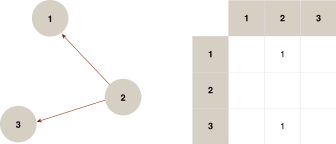# 1.0 - 8.00 - Centrality Calculation - Teradata Vantage

## Teradata® Vantage Machine Learning Engine Analytic Function Reference

Product
Release Number
1.0
8.00
Release Date
May 2019
Content Type
Programming Reference
Publication ID
B700-4003-098K
Language
English (United States)

To calculate centrality using the formulas described in Centrality Formulas, the EigenVectorCentrality function uses an in-neighbors relation matrix of the input source key and target key. In this matrix, aij has the value 1 if there is an edge from j to i.

In-Neighbors Relation MatrixIf you need an out-neighbors adjacent matrix—for example, to calculate the contribution of a vertex to other vertices—exchange the source key and target key columns and then invoke this function.